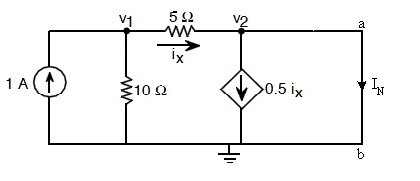# Thevenin and Norton Equivalent

## Homework Statement

For this problem (pic attached) I did the open circuit calculations and found V1 (6v) and V2 (4v) and I know they are correct. However I am not sure if my Voc and Isc (open circuit voltage and short circuit current) calculations are correct.

## The Attempt at a Solution

I am assuming V2 is Voc since they are in parallel? Also after creating a short circuit to find Isc, I used KCL at Node 1:

(V1/10)+(V1/5)=1
V1=3.33

So, Isc = V1/5ohms = 0.67 Amps (Because the current wouldn't flow through the branches with current dependent current source and 20ohms resistor)

Thanks for any help. Long time reader first time poster :)

#### Attachments

•Thevenin.jpg
8.2 KB · Views: 448

gneill
Mentor

## Homework Statement

For this problem (pic attached) I did the open circuit calculations and found V1 (6v) and V2 (4v) and I know they are correct. However I am not sure if my Voc and Isc (open circuit voltage and short circuit current) calculations are correct.

## The Attempt at a Solution

I am assuming V2 is Voc since they are in parallel? Also after creating a short circuit to find Isc, I used KCL at Node 1:

(V1/10)+(V1/5)=1
V1=3.33

So, Isc = V1/5ohms = 0.67 Amps (Because the current wouldn't flow through the branches with current dependent current source and 20ohms resistor)

Thanks for any help. Long time reader first time poster :)

Hi Tekneek, Welcome to Physics Forums.

Your calculations look fine. For the short circuit current calculation you could also have used the current divider formula, since the 1A divides between the 10 Ω and 5 Ω resistors.

Hi Tekneek, Welcome to Physics Forums.

Your calculations look fine. For the short circuit current calculation you could also have used the current divider formula, since the 1A divides between the 10 Ω and 5 Ω resistors.

Thanks for the reply. How would you get the current using the Voltage divider formula?

gneill
Mentor
Thanks for the reply. How would you get the current using the Voltage divider formula?

Current divider, not voltage divider.

•1 person
Current divider, not voltage divider.

Yeah got it. I = [(Add 10 and 5 in parallel)/5ohms]*1amp = 0.67 Amp

Thanks!

gneill
Mentor
Yeah got it. I = [(Add 10 and 5 in parallel)/5ohms]*1amp = 0.67 Amp

Thanks!

Whoops! I just realized that the controlled current source is still in the circuit even though the output is shorted! The path for the short circuit current is separate... so it'll look like:The controlled current source is going to "steal" half the current before it gets to where the short circuit is. Very sorry about that.